SBAS486F November   2009  – February 2016

PRODUCTION DATA.

1. Features
2. Applications
3. Description
4. Revision History
5. Pin Configuration and Functions
6. Specifications
7. Parameter Measurement Information
8. Detailed Description
1. 8.1 Overview
2. 8.2 Functional Block Diagram
3. 8.3 Feature Description
4. 8.4 Device Functional Modes
1. 8.4.1 Device Configuration
2. 8.4.2 Power-Down
5. 8.5 Programming
1. 8.5.1 Serial Interface
2. 8.5.2 Serial Register Readout
6. 8.6 Register Maps
9. Application and Implementation
1. 9.1 Application Information
10. 10Power Supply Recommendations
11. 11Layout
1. 11.1 Layout Guidelines
1. 11.1.1 Board Design Considerations
12. 12Device and Documentation Support
13. 13Mechanical, Packaging, and Orderable Information

• RGZ|48
• RGZ|48

## 6 Specifications

### 6.1 Absolute Maximum Ratings(1)

MIN MAX UNIT
Supply voltage range, AVDD –0.3 2.1 V
Supply voltage range, AVDD_BUF –0.3 3.9 V
Supply voltage range, DRVDD –0.3 2.1 V
Voltage between AGND and DRGND –0.3 0.3 V
Voltage between AVDD to DRVDD (when AVDD leads DRVDD) –2.4 2.4 V
Voltage between DRVDD to AVDD (when DRVDD leads AVDD) –2.4 2.4 V
Voltage between AVDD_BUF to DRVDD, AVDD –4.2 4.2 V
Voltage applied to input pins INP, INM –0.3 Minimum
(1.9, AVDD + 0.3)
V
CLKP, CLKM(2) –0.3 AVDD + 0.3
RESET, SCLK, SDATA, SEN, DFS –0.3 3.6
Temperature Operating free-air, TA –40 125 °C
Operating junction, TJ 150
Storage, Tstg –65 150
(1) Stresses beyond those listed under Absolute Maximum Ratings may cause permanent damage to the device. These are stress ratings only, which do not imply functional operation of the device at these or any other conditions beyond those indicated under Recommended Operating Conditions. Exposure to absolute-maximum-rated conditions for extended periods may affect device reliability.
(2) When AVDD is turned off, switching off the input clock (or ensuring the voltage on CLKP, CLKM is less than |0.3 V|) is recommended. Doing so prevents the ESD protection diodes at the clock input pins from turning on.

### 6.2 ESD Ratings

VALUE UNIT
V(ESD) Electrostatic discharge Human-body model (HBM), per ANSI/ESDA/JEDEC JS-001(1) ±2000 V
(1) JEDEC document JEP155 states that 500-V HBM allows safe manufacturing with a standard ESD control process.

### 6.3 Recommended Operating Conditions

MIN NOM MAX UNIT
SUPPLIES
AVDD Analog supply voltage 1.7 1.8 1.9 V
AVDD_BUF Analog buffer supply voltage 3 3.3 3.6 V
DRVDD Digital supply voltage 1.7 1.8 1.9 V
Differential input voltage range(2) 1.5 VPP
Input common-mode voltage 1.7 ± 0.05 V
Maximum analog input frequency with 1.5-VPP input amplitude(1) 400 MHz
Maximum analog input frequency with 1-VPP input amplitude(1) 600 MHz
CLOCK INPUT
Low-speed mode enabled(3) 20 80 MSPS
Low-speed mode disabled(3) > 80 250 MSPS
Input clock amplitude differential (VCLKP – VCLKM)
Sine wave, ac-coupled 0.2 1.5 VPP
LVPECL, ac-coupled 1.6 VPP
LVDS, ac-coupled 0.7 VPP
LVCMOS, single-ended, ac-coupled 1.8 V
Input clock duty cycle Low-speed mode enabled 40% 50% 60%
Low-speed mode disabled 35% 50% 65%
DIGITAL OUTPUTS
CLOAD Maximum external load capacitance from each output pin to DRGND 5 pF
RLOAD Differential load resistance between the LVDS output pairs (LVDS mode) 100 Ω
TJ Operating junction temperature Recommended 108 °C
Maximum rated(7) 125
(1) See the Overview section in the Detailed Description.
(2) With 0-dB gain. See the Gain for SFDR, SNR Trade-Off section in Feature Description for the relationship between input voltage range and gain.
(3) See the Serial Interface section for details on the low-speed mode.
(4) Using these modes is recommended to get best performance. These modes can only be set with the serial interface.
(5) See the Serial Interface section for details on register programming.
(6) Note that these modes cannot be set when the serial interface is not used (when the RESET pin is tied high); see the Device Configuration section.
(7) Prolonged use at this junction temperature can increase the device failure-in-time (FIT) rate.

### 6.4 Thermal Information

RGZ (VQFN)
48 PINS
RθJA Junction-to-ambient thermal resistance 27.9 °C/W
RθJC(top) Junction-to-case (top) thermal resistance 15.1 °C/W
RθJB Junction-to-board thermal resistance 5.4 °C/W
ψJT Junction-to-top characterization parameter 0.3 °C/W
ψJB Junction-to-board characterization parameter 5.4 °C/W
RθJC(bot) Junction-to-case (bottom) thermal resistance 1.7 °C/W
(1) For more information about traditional and new thermal metrics, see the Semiconductor and IC Package Thermal Metrics application report, SPRA953.

### 6.5 Electrical Characteristics: General

Typical values are at TA = 25°C, AVDD = 1.8 V, AVDD_BUF = 3.3 V, DRVDD = 1.8 V, and 50% clock duty cycle, unless otherwise noted. Minimum and maximum values are across the full ambient temperature range: TA, MIN = –40°C to TA, MAX = 85°C, AVDD = 1.8 V, AVDD_BUF = 3.3 V, and DRVDD = 1.8 V, unless otherwise noted.(3)
PARAMETER TEST CONDITIONS MIN TYP MAX UNIT
Differential input voltage range 1.5 VPP
Differential input resistance At dc (see Figure 83) 10
Differential input capacitance
(see Figure 84)
3.5 pF
(per input pin)
2 µA
VCM Common-mode output voltage 1.7 V
VCM output current capability 4 mA
DC ACCURACY
Offset error –15 2.5 15 mV
Temperature coefficient of offset error 0.003 mV/°C
EGREF Gain error as a result of
internal reference inaccuracy alone
–2 2 %FS
EGCHAN Gain error of channel alone 2.5 %FS
POWER SUPPLY
IAVDD Analog supply current 99.5 115 mA
IAVDD_BUF Analog input buffer supply current 29 42 mA
IDRVDD Output buffer supply current(2) LVDS interface with 100-Ω external termination, low LVDS swing (200 mV) 63 mA
LVDS interface with 100-Ω external termination, standard LVDS swing
(350 mV)
75 90
IDRVDD output buffer supply current(2)(1) CMOS interface(1), 8-pF external load capacitance, fIN = 2.5 MHz 35 mA
Global power-down 10 25 mW
Standby 200 mW
(1) In CMOS mode, the DRVDD current scales with the sampling frequency, the load capacitance on output pins, input frequency, and the supply voltage (see the CMOS Interface Power Dissipation section in the Feature Description).
(2) The maximum DRVDD current with CMOS interface depends on the actual load capacitance on the digital output lines. Note that the maximum recommended load capacitance on each digital output line is 10 pF.
(3) Minimum values for ADS41B49 are specified across the ambient temperature range of –40°C to +105°C.

Typical values are at TA = 25°C, AVDD = 1.8 V, AVDD_BUF = 3.3 V, DRVDD = 1.8 V, 1.5-VPP clock amplitude, 50% clock duty cycle, –1-dBFS differential analog input, and DDR LVDS interface, unless otherwise noted. Minimum and maximum values are across the full ambient temperature range: TA, MIN = –40°C to TA, MAX = 85°C, AVDD = 1.8 V, AVDD_BUF = 3.3 V, and DRVDD = 1.8 V, unless otherwise noted.
MIN TYP MAX MIN TYP MAX
Resolution 12 14 Bits
SNR Signal-to-noise ratio, LVDS fIN = 20 MHz 68.4 69.7 dBFS
fIN = 70 MHz 68.3 69.5
fIN = 100 MHz 68.3 69.5
fIN = 170 MHz 65.5 68 66.5 69.1
fIN = 300 MHz 67.5 68.4
SINAD Signal-to-noise and distortion ratio, LVDS fIN = 20 MHz 68.3 69.5 dBFS
fIN = 70 MHz 68.1 69.3
fIN = 100 MHz 68.2 69.3
fIN = 170 MHz 65 67.8 66 68.8
fIN = 300 MHz 66.5 67.4
SFDR Spurious-free dynamic range fIN = 20 MHz 89 89 dBc
fIN = 70 MHz 85 85
fIN = 100 MHz 87 87
fIN = 170 MHz 71 82 72 82
fIN = 300 MHz 75 75
THD Total harmonic distortion fIN = 20 MHz 85 85 dBc
fIN = 70 MHz 82 82
fIN = 100 MHz 83 83
fIN = 170 MHz 68 79.5 69 79.5
fIN = 300 MHz 72 72
HD2 Second-order harmonic distortion fIN = 20 MHz 93 93 dBc
fIN = 70 MHz 85 85
fIN = 100 MHz 87 87
fIN = 170 MHz 71 87 72 87
fIN = 300 MHz 80 80
HD3 Third-order harmonic distortion fIN = 20 MHz 93 93 dBc
fIN = 70 MHz 88 88
fIN = 100 MHz 88 88
fIN = 170 MHz 71 82 72 82
fIN = 300 MHz 75 75
Worst spur
(other than second- and third-order harmonics)
fIN = 20 MHz 89 89 dBc
fIN = 70 MHz 90 90
fIN = 100 MHz 90 90
fIN = 170 MHz 76 88 77.5 88
fIN = 300 MHz 88 88
IMD Two-tone intermodulation distortion f1 = 185 MHz, f2 = 190 MHz,
each tone at –7 dBFS
–86 –86 dBFS
Input overload recovery Recovery to within 1% (of final value) for 6-dB overload with sine-wave input 1 1 Clock cycles
PSRR AC power-supply rejection ratio For 100-mVPP signal on AVDD supply, up to 10 MHz > 30 > 30 dB
ENOB Effective number of bits fIN = 170 MHz 11 11.2 LSBs
INL Integrated nonlinearity fIN = 170 MHz ±1.5 ±3.5 ±2.5 ±5 LSBs
(1) Minimum values for the ADS41B49 are specified across the ambient temperature range of –40°C to +105°C.

### 6.7 Digital Characteristics(4)

Typical values are at TA = 25°C, AVDD = 1.8 V, AVDD_BUF = 3.3 V, and DRVDD = 1.8 V, unless otherwise noted. Minimum and maximum values are across the full ambient temperature range: TA, MIN = –40°C to TA, MAX = 85°C, AVDD = 1.8 V, AVDD_BUF = 3.3 V, and DRVDD = 1.8 V.
PARAMETER TEST CONDITIONS MIN TYP MAX UNIT
DIGITAL INPUTS (RESET, SCLK, SDATA, SEN, OE)
High-level input voltage RESET, SCLK, SDATA, and SEN support 1.8-V and 3.3-V CMOS logic levels 1.3 V
OE only supports 1.8-V CMOS logic levels 1.3 V
Low-level input voltage RESET, SCLK, SDATA, and SEN support 1.8-V and 3.3-V CMOS logic levels 0.4 V
OE only supports 1.8-V CMOS logic levels 0.4 V
High-level input current SDATA, SCLK(1) VHIGH = 1.8 V 10 µA
SEN(3) VHIGH = 1.8 V 0 µA
Low-level input current SDATA, SCLK VLOW = 0 V 0 µA
SEN VLOW = 0 V –10 µA
DIGITAL OUTPUTS (CMOS INTERFACE: D0 TO D13, OVR_SDOUT)
High-level output voltage DRVDD – 0.1 DRVDD V
Low-level output voltage 0 0.1 V
DIGITAL OUTPUTS (LVDS INTERFACE: D0_D1_P/M to D12_D13_P/M, CLKOUTP/M)
VODH High-level output voltage(2) Standard swing LVDS 270 350 430 mV
Low swing LVDS 200 mV
VODL Low-level output voltage(2) Standard swing LVDS –430 –350 –270 mV
Low swing LVDS –200 mV
VOCM Output common-mode voltage 0.85 1.05 1.25 V
(1) SDATA and SCLK have an internal 180-kΩ pull-down resistor.
(2) With an external 100-Ω termination.
(3) SEN has an internal 180-kΩ pull-up resistor to AVDD.
(4) Minimum values for ADS41B49 are specified across the ambient temperature range of –40°C to +105°C.

### 6.8 Timing Requirements: LVDS and CMOS Modes

Typical values are at TA = 25°C, AVDD = 1.8 V, AVDD_BUF = 3.3 V, DRVDD = 1.8 V, sampling frequency = 250 MSPS, sine-wave input clock, CLOAD = 5 pF(2), and RLOAD = 100 Ω(3), unless otherwise noted. Minimum and maximum values are across the full ambient temperature range: TA, MIN = –40°C to TA, MAX = 85°C, AVDD = 1.8 V, AVDD_BUF = 3.3 V, and DRVDD = 1.7 V to 1.9 V.(1)
MIN TYP MAX UNIT
GENERAL
tA Aperture delay 0.6 0.8 1.2 ns
Variation of aperture delay between two devices at the same temperature and DRVDD supply ±100 ps
tJ Aperture jitter 100 fS rms
Wakeup time Time to valid data after coming out of STANDBY mode 5 25 µs
Time to valid data after coming out of PDN GLOBAL mode 100 500
ADC latency(6) Gain enabled (default after reset) 21 Clock cycles
Gain and offset correction enabled 22
DDR LVDS MODE
tSU Data setup time(3): data valid(4) to zero-crossing of CLKOUTP 0.75(7) 1.1 ns
tH Data hold time(3): zero-crossing of CLKOUTP to data becoming invalid(4) 0.35(8) 0.6 ns
tPDI Clock propagation delay: input clock rising edge cross-over to output clock rising edge cross-over, 1 MSPS ≤ sampling frequency ≤ 250 MSPS 3 4.2 5.4 ns
Variation of tPDI between two devices at the same temperature and DRVDD supply ±0.6 ns
LVDS bit clock duty cycle of differential clock, (CLKOUTP – CLKOUTM),
1 MSPS ≤ sampling frequency ≤ 250 MSPS
42% 48% 54%
tRISE, tFALL Data rise and fall time: rise time measured from –100 mV to +100 mV, fall time measured from +100 mV to –100 mV, 1 MSPS ≤ sampling frequency ≤ 250 MSPS 0.14 ns
tCLKRISE,
tCLKFALL
Output clock rise and fall time: rise time measured from –100 mV to +100 mV, fall time measured from +100 mV to –100 mV, 1 MSPS ≤ sampling frequency ≤ 250 MSPS 0.14 ns
tOE Output enable (OE) to data delay time to valid data after OE becomes active 50 100 ns
PARALLEL CMOS MODE(5)
tSTART Input clock to data delay: input clock rising edge cross-over to start of data valid(4) 1.6 ns
tDV Data valid time interval of valid data(4) 2.5 3.2 ns
tPDI Clock propagation delay: input clock rising edge cross-over to, output clock rising edge cross-over, 1 MSPS ≤ sampling frequency ≤ 200 MSPS 4 5.5 7 ns
Output clock duty cycle of output clock (CLKOUT),
1 MSPS ≤ sampling frequency ≤ 200 MSPS
47%
tRISE, tFALL Data rise and fall time: rise time measured from 20% to 80% of DRVDD, fall time measured from 80% to 20% of DRVDD, 1 MSPS ≤ sampling frequency ≤ 250 MSPS 0.35 ns
tCLKRISE,
tCLKFALL
Output clock rise and fall time: rise time measured from 20% to 80% of DRVDD, fall time measured from 80% to 20% of DRVDD,
1 MSPS ≤ sampling frequency ≤ 200 MSPS
0.35 ns
tOE Output enable (OE) to data delay time to valid data after OE becomes active 20 40 ns
(1) Timing parameters are ensured by design and characterization but are not production tested.
(2) CLOAD is the effective external single-ended load capacitance between each output pin and ground.
(3) RLOAD is the differential load resistance between the LVDS output pair.
(4) Data valid refers to a logic high of 1.26 V and a logic low of 0.54 V.
(5) For fS > 200 MSPS, using an external clock is recommended for data capture instead of the device output clock signal (CLKOUT).
(6) At higher frequencies, tPDI is greater than one clock period and overall latency = ADC latency + 1.
(7) For an ambient temperature range of –40°C to +105°C, the minimum value of setup time reduces to 0.7 ns.
(8) For an ambient temperature range of –40°C to +105°C, the minimum value of setup time reduces to 0.3 ns.

### 6.9 Timing Requirements: Reset

Typical values at TA = 25°C and minimum and maximum values across the full ambient temperature range: TA, MIN = –40°C to TA, MAX = 85°C, unless otherwise noted.(2)
MIN TYP MAX UNIT
t1 Power-on delay from power-up of AVDD and DRVDD to RESET pulse active 1 ms
t2 Reset pulse duration of active RESET signal that resets the serial registers 10 ns
1(1) µs
t3 Delay from RESET disable to SEN active 100 ns
(1) The reset pulse is needed only when using the serial interface configuration. If the pulse width is greater than 1 µs, the device could enter the parallel configuration mode briefly and then return back to serial interface mode.
(2) For the ADS41B49, the minimum and maximum values are given for the ambient temperature range of TA, MIN = –40°C to TA, MAX = 105°C.

### 6.10 Timing Requirements: LVDS Timing Across Sampling Frequencies

SAMPLING FREQUENCY (MSPS) SETUP TIME (ns) HOLD TIME (ns)
MIN TYP MAX MIN TYP MAX
230 0.85 1.25 0.35 0.6
200 1.05 1.55 0.35 0.6
185 1.1 1.7 0.35 0.6
160 1.6 2.1 0.35 0.6
125 2.3 3 0.35 0.6
80 4.5 5.2 0.35 0.6

### 6.11 Timing Requirements: CMOS Timing Across Sampling Frequencies

SAMPLING FREQUENCY (MSPS) TIMING SPECIFIED WITH RESPECT TO OUTPUT CLOCK
tSETUP (ns) tHOLD (ns) tPDI (ns)
MIN TYP MAX MIN TYP MAX MIN TYP MAX
200 1 1.6 2 2.8 4 5.5 7
185 1.3 2 2.2 3 4 5.5 7
160 1.8 2.5 2.5 3.3 4 5.5 7
125 2.5 3.2 3.5 4.3 4 5.5 7
80 4.8 5.5 5.7 6.5 4 5.5 7

### 6.12 Timing Requirements: CMOS Timing Across Sampling Frequencies

SAMPLING FREQUENCY (MSPS) TIMING SPECIFIED WITH RESPECT TO INPUT CLOCK
tSTART (ns) tDV (ns)
MIN TYP MAX MIN TYP MAX
250 1.6 2.5 3.2
230 1.1 2.9 3.5
200 0.3 3.5 4.2
185 0 3.9 4.5
170 –1.3 4.3 5

### 6.13 Typical Characteristics: ADS41B49

At 25°C, AVDD = 1.8 V, AVDD_BUF = 3.3 V, DRVDD = 1.8 V, maximum-rated sampling frequency, sine wave input clock, 1.5-VPP differential clock amplitude, 50% clock duty cycle, –1-dBFS differential analog input, DDR LVDS output interface, and 32k-point FFT, unless otherwise noted.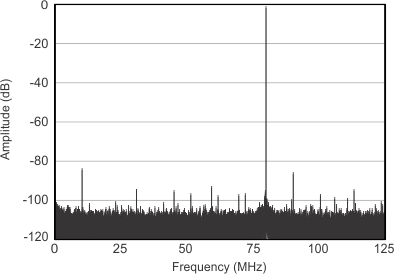SFDR = 90.3 dBc, SNR = 69.9 dBFS, SINAD = 69.8 dBFS, THD = 85.2 dBc
Figure 1. FFT for 20-MHz Input Signal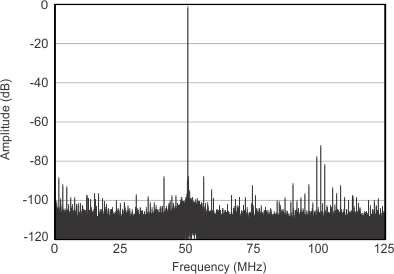SFDR = 70.7 dBc, SNR = 68.4 dBFS, SINAD = 66.3 dBFS, THD = 69.3 dBc
Figure 3. FFT for 300-MHz Input Signal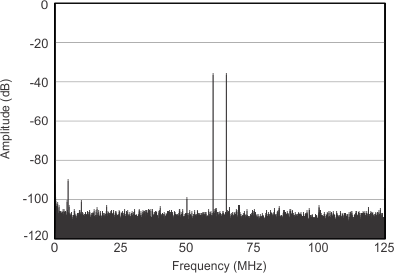Each tone at –36-dBFS amplitude, fIN1 = 185 MHz, fIN2 = 190 MHz, two-tone IMD = 89.7 dBFS, SFDR = 106.4 dBFS
Figure 5. FFT for Two-Tone Input Signal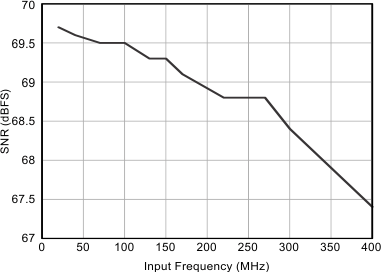Figure 7. SNR vs Input Frequency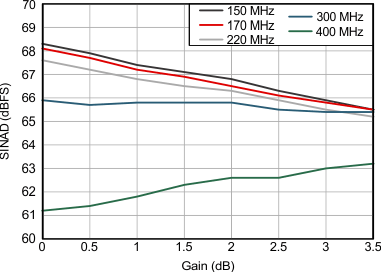Figure 9. SINAD Across Gain and Input Frequency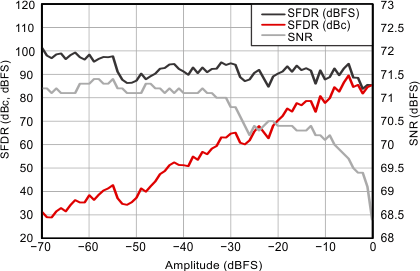Input frequency = 170 MHz
Figure 11. Performance Across Input Amplitude
(Single Tone)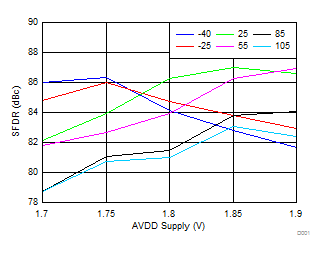Input frequency = 170 MHz
Figure 13. SFDR Across Ambient Temperature vs AVDD SupplyInput frequency = 170 MHz
Figure 15. Performance Across DRVDD Supply Voltage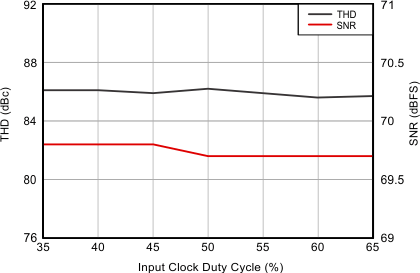Input frequency = 10 MHz
Figure 17. Performance Across Input Clock Duty Cycle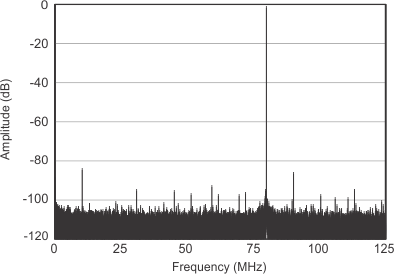SFDR = 82.9 dBc, SNR = 69.3 dBFS, SINAD = 69 dBFS, THD = 80.3 dBc
Figure 2. FFT for 170-MHz Input Signal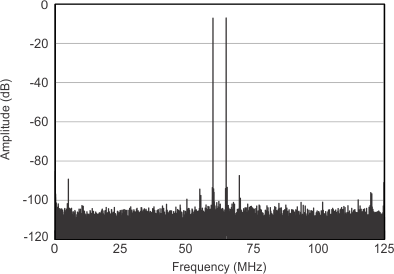Each tone at –7-dBFS amplitude, fIN1 = 185 MHz, fIN2 = 190 MHz, two-tone IMD = 87.3 dBFS, SFDR = 96.0 dBFS
Figure 4. FFT for Two-Tone Input Signal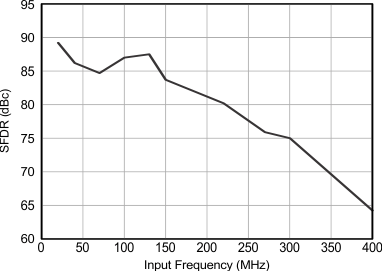Figure 6. SFDR vs Input Frequency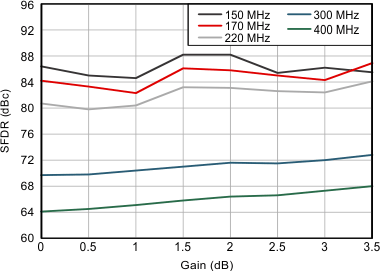Figure 8. SFDR Across Gain and Input Frequency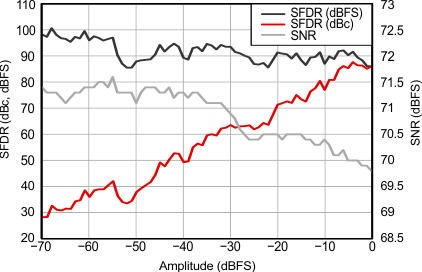Input frequency = 40 MHz
Figure 10. Performance Across Input Amplitude
(Single Tone)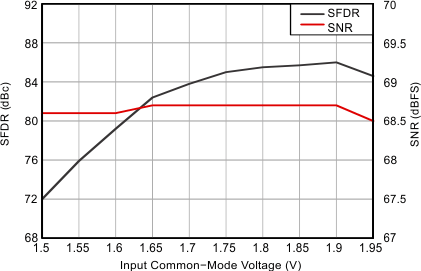Input frequency = 170 MHz
Figure 12. Performance vs Input Common-Mode Voltage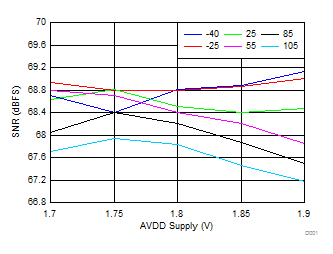Input frequency = 170 MHz
Figure 14. SNR Across Ambient Temperature vs AVDD Supply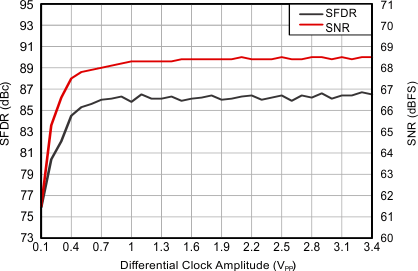Input frequency = 170 MHz
Figure 16. Performance Across Input Clock Amplitude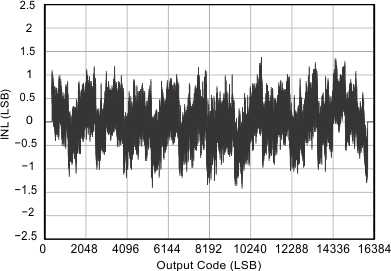Figure 18. Integral Nonlinearity

### 6.14 Typical Characteristics: ADS41B29

At 25°C, AVDD = 1.8 V, AVDD_BUF = 3.3 V, DRVDD = 1.8 V, maximum-rated sampling frequency, sine wave input clock, 1.5-VPP differential clock amplitude, 50% clock duty cycle, –1-dBFS differential analog input, DDR LVDS output interface, and 32k-point FFT, unless otherwise noted.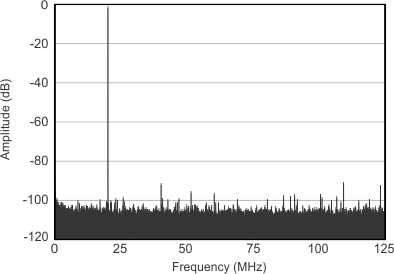SFDR = 89.6 dBc, SNR = 68.6 dBFS, SINAD = 68.5 dBFS, THD = 85.2 dBc
Figure 19. FFT for 20-MHz Input Signal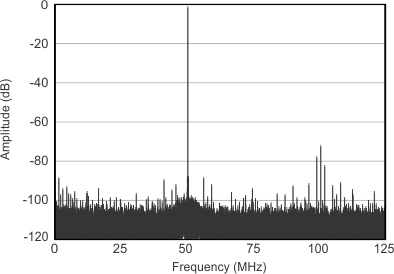SFDR = 70.8 dBc, SNR = 67.4 dBFS, SINAD = 65.7 dBFS, THD = 69.4 dBc
Figure 21. FFT for 300-MHz Input Signal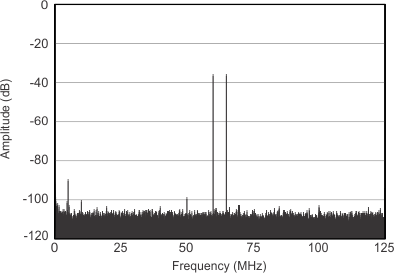Each tone at –36-dBFS amplitude, fIN1 = 185 MHz, fIN2 = 190 MHz, two-tone IMD = 89.8 dBFS, SFDR = 98.5 dBFS
Figure 23. FFT for Two-Tone Input SignalFigure 25. SNR vs Input Frequency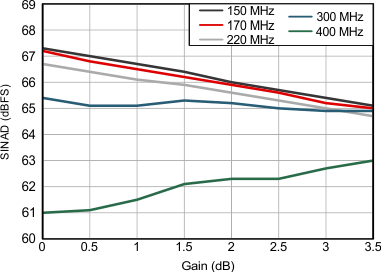Figure 27. SINAD Across Gain and Input Frequency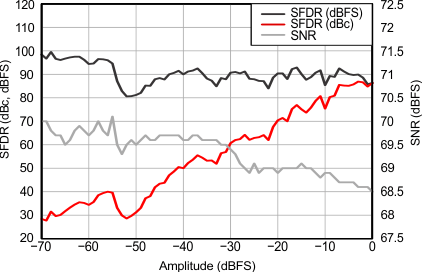Input frequency = 40 MHz
Figure 29. Performance Across Input Amplitude
(Single Tone)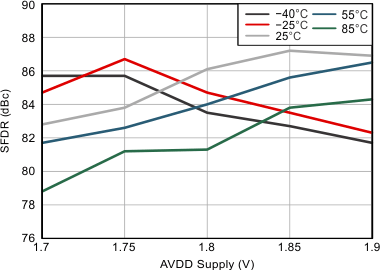Input frequency = 170 MHz
Figure 31. SFDR Across Ambient Temperature vs AVDD Supply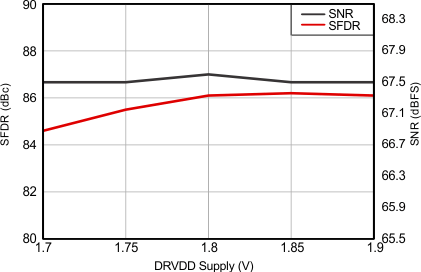Input frequency = 170 MHz
Figure 33. Performance Across DRVDD Supply Voltage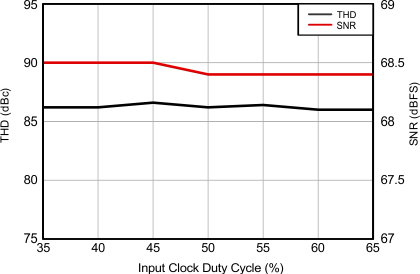Input frequency = 10 MHz
Figure 35. Performance Across Input Clock Duty Cycle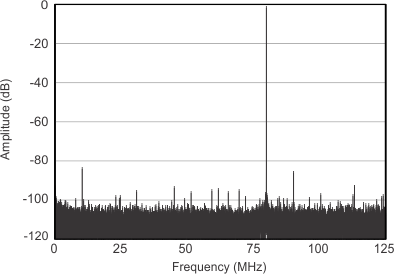SFDR = 82.3 dBc, SNR = 68.1 dBFS, SINAD = 67.8 dBFS, THD = 79.9 dBc
Figure 20. FFT for 170-MHz Input Signal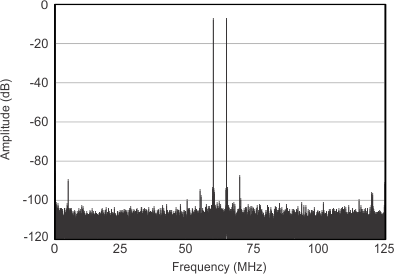Each tone at –7-dBFS amplitude, fIN1 = 185 MHz, fIN2 = 190 MHz, two-tone IMD = 87.3 dBFS, SFDR = 85.9 dBFS
Figure 22. FFT for Two-Tone Input Signal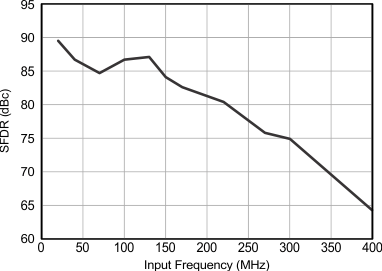Figure 24. SFDR vs Input Frequency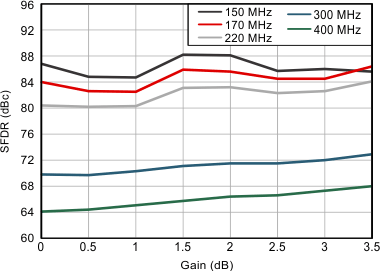Figure 26. SFDR Across Gain and Input Frequency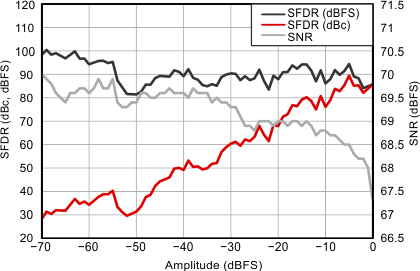Input frequency = 170 MHz
Figure 28. Performance Across Input Amplitude
(Single Tone)Input frequency = 170 MHz
Figure 30. Performance vs input Common-Mode VoltageInput frequency = 170 MHz
Figure 32. SNR Across Ambient Temperature vs AVDD Supply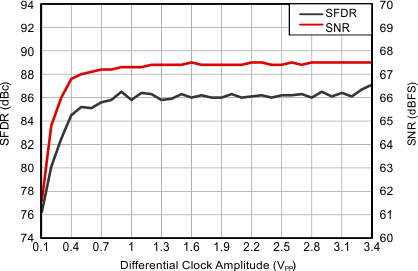Input frequency = 170 MHz
Figure 34. Performance Across Input Clock Amplitude

### 6.15 Typical Characteristics: General

At 25°C, AVDD = 1.8 V, AVDD_BUF = 3.3 V, DRVDD = 1.8 V, maximum-rated sampling frequency, sine wave input clock, 1.5-VPP differential clock amplitude, 50% clock duty cycle, –1-dBFS differential analog input, DDR LVDS output interface, and 32k-point FFT, unless otherwise noted.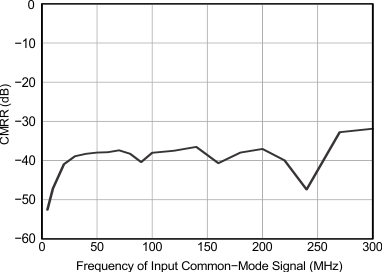Input frequency = 170 MHz, 50-mVPP signal superimposed on input common-mode voltage (1.7 V)
Figure 36. CMRR Across FrequencyFigure 38. PSRR Across Frequency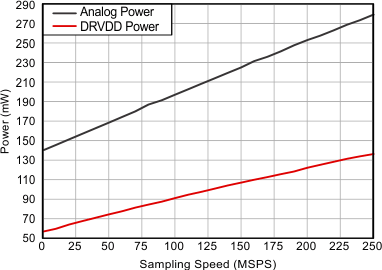Figure 40. Power Across Sampling Frequency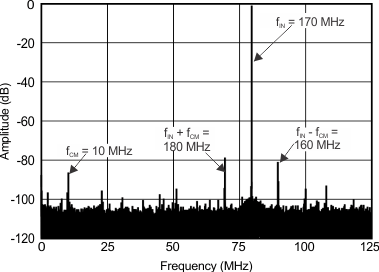fIN = 170 MHz; fCM = 10 MHz, 50 mVPP; SFDR = 77.69 dB; amplitude: (fIN) = –1 dBFS; (fCM) = –93.8 dBFS; (fIN + fCM) = –78.8 dBFS; (fIN – fCM) = –81 dBFS
Figure 37. CMRR FFT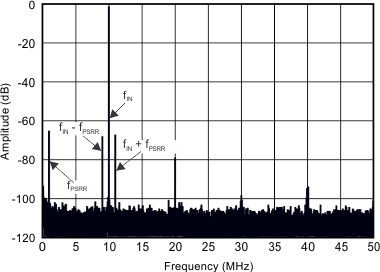fIN = 10 MHz; fPSRR = 10 MHz, 50 mVPP; amplitude: (fIN) = –1 dBFS; (fPSRR) = –65.6 dBFS; (fIN + fPSRR) = –67.5 dBFS; (fIN – fPSRR) = –68.3 dBFS
Figure 39. PSRR FFT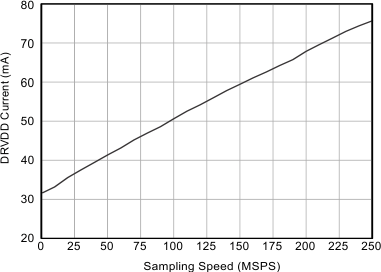Figure 41. DRVDD Current Across Sampling Frequency

### 6.16 Typical Characteristics: Contour

At 25°C, AVDD = 1.8 V, AVDD_BUF = 3.3 V, DRVDD = 1.8 V, maximum-rated sampling frequency, sine wave input clock, 1.5-VPP differential clock amplitude, 50% clock duty cycle, –1-dBFS differential analog input, DDR LVDS output interface, and 32k-point FFT, unless otherwise noted.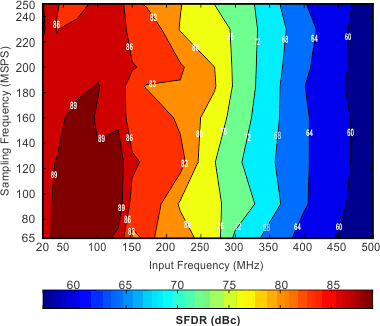Figure 42. SFDR Contour
(0-dB Gain, Applies to ADS41Bx9)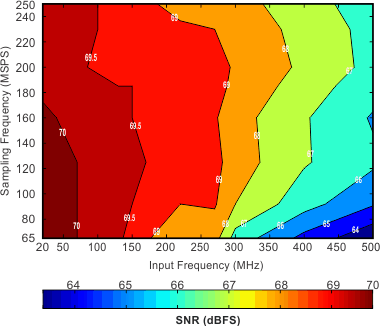Figure 44. SNR Contour
(0-dB Gain, Applies to ADS41B49)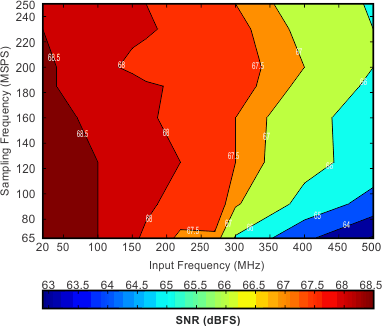Figure 46. SNR Contour
(0-dB Gain, Applies to ADS41B29)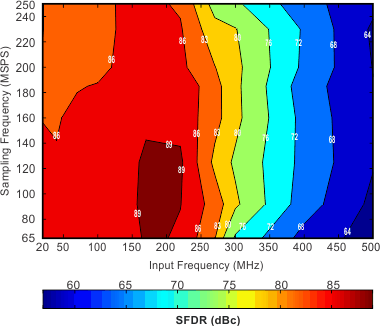Figure 43. SFDR Contour
(3.5-dB Gain, Applies to ADS41Bx9)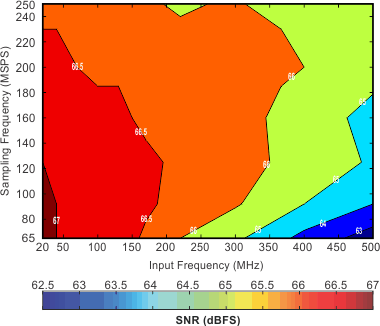Figure 45. SNR Contour
(3.5-dB Gain, Applies to ADS41B49)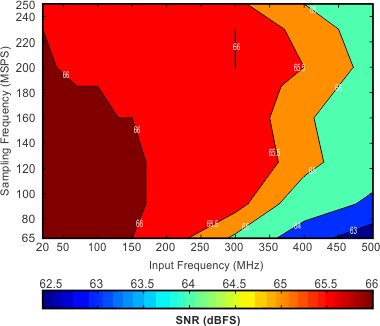Figure 47. SNR Contour
(0-dB Gain, Applies to ADS41B29)